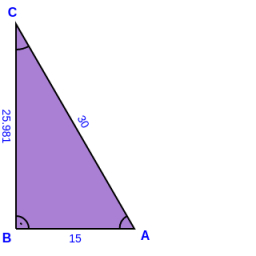# Calculate 60993

In the right triangle ABC, calculate the magnitude of the interior angles if / AB / = 13 cm; / BC / = 12 cm and / AC / = 5 cm.

γ =  90 °
α =  67.3801 °
β =  22.6199 °

### Step-by-step explanation:

Try calculation via our triangle calculator.Did you find an error or inaccuracy? Feel free to write us. Thank you!

Tips for related online calculators